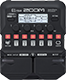# Slipknot

Discussion in 'Zoom G1/G1X Four' started by Charmer, Apr 18, 2021.

1. SlipknotDevice: Zoom G1 Four
Firmware: 2.00

Name on device: SlipKDST
Optimized for: Guitar Amp

Effects chain:For active pickaps (18 V MOD).

Effect: "ZNR" (Dynamics), active - "yes"
"Detect" = EFXIN
"Depth" = 59
"Threshold" = 10
"Decay" = 35

Effect: "MetalWRLD" (Overdrive / Distortion), active - "yes"
"Gain" = 100
"Bass" = 65
"Treble" = 86
"Volume" = 100

Effect: "Gt GEQ 7" (Filter), active - "yes"
"100" = -1.5
"200" = -1.0
"400" = -1.5
"800" = -3.0
"1.6k" = -2.0
"3.2k" = -0.5
"6.4k" = -6.0
"VOL" = 100

Effect: "ParaEQ" (Filter), active - "yes"
"Frequency" = 10.0kHz
"Q" = 4.6
"Gain" = 12.0
"Volume" = 83

Effect: "ORG4x12" (Cabinet), active - "yes"
"MIC" = ON
"D57: D421" = 62
"Hi" = 46
"Lo" = 55

Patch Volume: 64